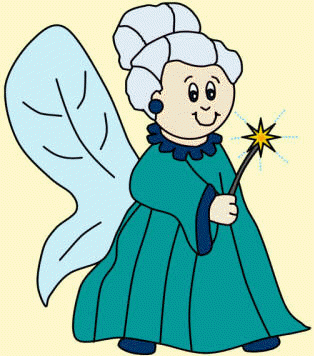# KidZone.ws Mathematical Magic Trick

Appropriate for Grades 3 and up  (people who know their 2 times tables and 5 times tables)

Impress your friends and give them a little practice with their math times tables with this mathematical magic trick.Tell your friend to pick their favorite number between 1 and 100, but don't tell you what it is! Note:  If your friends are children, have them pick a number between 1 and 9 so they are able to do the next steps.  If you're doing this with mom and dad, you can tease them and make them pick REALLY big numbers so they have to work hard at the math.  They'll be very surprised when you come back with the answer so quickly! Have them multiply their number by 2. Have them multiply their number by 5. Have them tell you the answer they've come up with. Drop the zero from the answer they gave you and tell them what their number was!

#### Example 1:

Favorite number = 5

5 x 2 = 10

10 x 5 = 50

50 --- drop the zero = 5!

#### Example 2:

Favorite number = 32

32 x 2 = 64

64 x 5 = 320

320 --- drop the zero = 32!

How it works:  2 x 5 = 10 so really, you're having them multiply their number by 10 ... which is just the same as having them add a zero to the end of their number.  But by having them do this in two stages (first multiply by 2, then multiply by 5), people beginning with multiplication won't realize this right away.  They'll either think you're reading their mind or that you're very fast at dividing.  Eventually, they may realize that it's the math that's magical, not you!